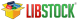`ANSI C Stdlib Library  mikroC PRO for PIC32 Libraries > Standard ANSI C Libraries > ` `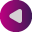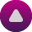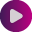`

## ANSI C Stdlib Library

The mikroC PRO for PIC32 provides a set of standard ANSI C library functions of general utility.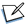Important :
• Not all of the standard functions have been included.
• The functions have been mostly implemented according to the ANSI C standard, but certain functions have been modified in order to facilitate PIC32 programming. Be sure to skim through the description before using standard C functions.

### Library Dependency Tree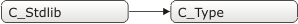### abs

Prototype int abs(int a); Function returns the absolute (i.e. positive) value of `a`. `result = abs(-12); // result = 12`

### atof

Prototype double atof(char *s); Function converts the input string `s` into a double precision value and returns the value. Input string `s` should conform to the floating point literal format, with an optional whitespace at the beginning. The string will be processed one character at a time, until the function reaches a character which it doesn’t recognize (including a null character). `doub = atof("-1.23"); // doub = -1.23`

### atoi

Prototype int atoi(char *s); Function converts the input string `s` into an integer value and returns the value. The input string `s` should consist exclusively of decimal digits, with an optional whitespace and a sign at the beginning. The string will be processed one character at a time, until the function reaches a character which it doesn’t recognize (including a null character). `result = atoi("32000"); // result = 32000`

### atol

Prototype long atol(char *s); Function converts the input string `s` into a long integer value and returns the value. The input string `s` should consist exclusively of decimal digits, with an optional whitespace and a sign at the beginning. The string will be processed one character at a time, until the function reaches a character which it doesn’t recognize (including a null character). `result = atol("-32560"); // result = -32560`

### div

Prototype div_t div(int number, int denom); Function computes the result of division of the numerator `number` by the denominator `denom`; the function returns a structure of type `div_t` comprising quotient (`quot`) and remainder (`rem`), see Div Structures. `dt = div(1234,100);`

### ldiv

Prototype ldiv_t ldiv(long number, long denom); Function is similar to the div function, except that the arguments and result structure members all have type `long`. Function computes the result of division of the numerator `number` by the denominator `denom`; the function returns a structure of type `ldiv_t` comprising quotient (`quot`) and remainder (`rem`), see Div Structures. `dl = ldiv(-123456, 1000);`

### uldiv

Prototype uldiv_t uldiv(unsigned long number, unsigned long denom); Function is similar to the div function, except that the arguments and result structure members all have type `unsigned long`. Function computes the result of division of the numerator `number` by the denominator `denom`; the function returns a structure of type `uldiv_t` comprising quotient (`quot`) and remainder (`rem`), see Div Structures. `dul = uldiv(123456,1000);`

### labs

Prototype long labs(long x); Function returns the absolute (i.e. positive) value of long integer `x`. `result = labs(-2147483647);`

### max

Prototype int max(int a, int b); Function returns greater of the two integers, `a` and `b`. `result = max(123,67); // function returns 123`

### min

Prototype int min(int a, int b); Function returns lower of the two integers, `a` and `b`. `result = min(123,67); // function returns 67`

### rand

Prototype int rand(); Function returns a sequence of pseudo-random numbers between 0 and 32767. The function will always produce the same sequence of numbers unless srand is called to seed the start point. ```while(1) result = rand() ;```

### srand

Prototype void srand(unsigned x); Function uses `x` as a starting point for a new sequence of pseudo-random numbers to be returned by subsequent calls to rand. No values are returned by this function. `srand(9);`

### xtoi

Prototype unsigned xtoi(char *s); Function converts the input string `s` consisting of hexadecimal digits into an integer value. The input parameter `s` should consist exclusively of hexadecimal digits, with an optional whitespace and a sign at the beginning. The string will be processed one character at a time, until the function reaches a character which it doesn’t recognize (including a null character). `result = xtoi("1FF"); // result = 511`

#### Div Structures

``` typedef struct divstruct {
int quot;
int rem;
} div_t;

typedef struct ldivstruct {
long quot;
long rem;
} ldiv_t;

typedef struct uldivstruct {
unsigned long quot;
unsigned long rem;
} uldiv_t;
```
Find them on